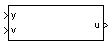# 1D Controller [A(v),B(v),C(v),D(v)]

Implement gain-scheduled state-space controller depending on one scheduling parameter

•Libraries:
Aerospace Blockset / GNC / Control

## Description

The 1D Controller [A(v),B(v),C(v),D(v)] block implements a gain-scheduled state-space controller, as described in Algorithms.

The output from this block is the actuator demand, which you can input to an actuator block.

## Limitations

If the scheduling parameter inputs to the block go out of range, they are clipped. The state-space matrices are not interpolated out of range.

## Ports

### Input

expand all

Aircraft measurements, specified as a vector.

Data Types: `double`

Scheduling variable, specified as a vector, that conforms to the dimensions of the state-space matrices.

Data Types: `double`

### Output

expand all

Actuator demands, specified as a vector.

Data Types: `double`

## Parameters

expand all

A-matrix of the state-space implementation, specified as a array. In the case of 1-D scheduling, the A-matrix should have three dimensions, the last one corresponding to the scheduling variable v. For example, if the A-matrix corresponding to the first entry of v is the identity matrix, then `A(:,:,1) = [1 0;0 1];`.

#### Programmatic Use

 Block Parameter: `A` Type: character vector Values: vector Default: `'A1'`

B-matrix of the state-space implementation, specified as a array. In the case of 1-D scheduling, the B-matrix should have three dimensions, the last one corresponding to the scheduling variable v. For example, if the B-matrix corresponding to the first entry of v is the identity matrix, then ```B(:,:,1) = [1 0;0 1];```.

#### Programmatic Use

 Block Parameter: `B` Type: character vector Values: vector Default: `'B1'`

C-matrix of the state-space implementation, specified as a vector. In the case of 1-D scheduling, the C-matrix should have three dimensions, the last one corresponding to the scheduling variable v. For example, if the C-matrix corresponding to the first entry of v is the identity matrix, then ```C(:,:,1) = [1 0;0 1];```.

#### Programmatic Use

 Block Parameter: `C` Type: character vector Values: vector Default: `'C1'`

D-matrix of the state-space implementation, specified as a array. In the case of 1-D scheduling, the D-matrix should have three dimensions, the last one corresponding to the scheduling variable v. For example, if the D-matrix corresponding to the first entry of v is the identity matrix, then `D(:,:,1) = [1 0;0 1];`.

#### Programmatic Use

 Block Parameter: `D` Type: character vector Values: vector Default: `'D1'`

Breakpoints for the scheduling variable, specified as a vector. The length of v must be the same as the size of the third dimension of A, B, C, and D.

#### Programmatic Use

 Block Parameter: `AoA_vec` Type: character vector Values: vector Default: `'v_vec'`

Initial states for the controller, such as initial values for the state vector, x, specified as a vector. The length of the vector must equal the size of the first dimension of A.

#### Programmatic Use

 Block Parameter: `x_initial` Type: character vector Values: vector Default: `'0'`

## Algorithms

The block implements a gain-scheduled state-space controller as defined by this equation:

`$\begin{array}{l}\stackrel{˙}{x}=A\left(v\right)x+B\left(v\right)y\\ u=C\left(v\right)x+D\left(v\right)y\end{array}$`

where v is a parameter over which A, B, C, and D are defined. This type of controller scheduling assumes that the matrices A, B, C, and D vary smoothly as a function of v, which is often the case in aerospace applications.

## Version History

Introduced before R2006a